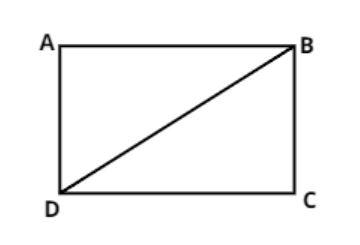QUESTION

# The diagonal of a rectangular field is 60 meters more than the shorter side. If the longer side is 30 meters more than the shorter side, find the sides of the field.

Hint:Let the shorter side be x m and the longer side be y m. Use the constraints given in questions along with the concept that the diagonal BD( see the figure) will divide the rectangle into two right angled triangles. Thus apply Pythagoras theorem to formulate relations between the two variables.Let ABCD be a rectangular field.
Let the shorter side of the rectangle be x meters.
And the longer side be y meters.
Therefore,
BC = x meter and DC = y meter.
Now it is given that the diagonal of a rectangular field is 60 meters more than the shorter side.
$\Rightarrow BD = 60 + BC$
$\Rightarrow BD = 60 + x$ meters.
Now it is also given that the longer side of a rectangular field is 30 meters more than the shorter side.
$\Rightarrow DC = 30 + BC$
$\Rightarrow y = 30 + x$............................ (1)
Now as we know that in a rectangle adjacent sides are perpendicular to each other therefore apply Pythagoras theorem in triangle BDC we have,
$\Rightarrow {\left( {{\text{Hypotenuse}}} \right)^2} = {\left( {{\text{perpendicular}}} \right)^2} + {\left( {{\text{base}}} \right)^2}$
$\Rightarrow {\left( {BD} \right)^2} = {\left( {BC} \right)^2} + {\left( {CD} \right)^2}$
Now substitute all the values in this equation we have,
$\Rightarrow {\left( {60 + x} \right)^2} = {\left( x \right)^2} + {\left( y \right)^2}$
Now from equation (1) we have,
$\Rightarrow {\left( {60 + x} \right)^2} = {\left( x \right)^2} + {\left( {30 + x} \right)^2}$
$\Rightarrow {x^2} = {\left( {60 + x} \right)^2} - {\left( {30 + x} \right)^2}$
Now apply the identity ${a^2} - {b^2} = \left( {a - b} \right)\left( {a + b} \right)$ we have,
$\Rightarrow {x^2} = \left( {60 + x - 30 - x} \right)\left( {60 + x + 30 + x} \right)$
$\Rightarrow {x^2} = \left( {30} \right)\left( {90 + 2x} \right)$
$\Rightarrow {x^2} = 60x + 2700$
$\Rightarrow {x^2} - 60x - 2700 = 0$
Now factorize this equation we have,
$\Rightarrow {x^2} - 90x + 30x - 2700 = 0$
$\Rightarrow x\left( {x - 90} \right) + 30\left( {x - 90} \right) = 0$
$\Rightarrow \left( {x - 90} \right)\left( {x + 30} \right) = 0$
$\Rightarrow x = 90, - 30$
As we know side cannot be in negative,
Therefore x = 90 meter is the valid case.
So the length of the shorter side = 90 meters.
And the length of the longer side = (30 + 90) = 120 meters.
So this is the required answer.

Note – A rectangle is a quadrilateral, such that the opposite sides are parallel and equal to each other. Each interior angle is equal to ${90^0}$, and the diagonals also bisect each other. In a rectangle the diagonals are congruent however diagonals are not perpendicular. The adjacent angles are supplementary too.